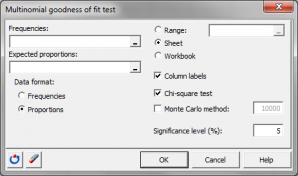# Multinomial goodness of fit test

Multinomial goodness of fit tests compare frequencies of the levels of a qualitative variable to theoretical frequencies. In Excel using the XLSTAT software.## What is the multinomial goodness of fit test

The multinomial goodness of fit test allows verifying whether the distribution of a sample corresponding to a qualitative variable (or discretized quantitative variable) is consistent with what is expected. The test is based on the multinomial distribution which is the extension of the binomial distribution when there are more than two possible outcomes.

## Multinomial goodness of fit test definition

Let k be the number of possible values (categories) for variable X. We write p1, p2, …, pk the probabilities (or densities) corresponding to each value.

Let n1, n2, n3, …, nk be the frequencies of each value for a sample.

• The null hypothesis of the test writes: H0: The distribution of the values in the sample is consistent with what is expected, meaning that the distribution of the sample is not different from the distribution of X.
• The alternative hypothesis of the test writes: Ha: The distribution of the values in the sample is not consistent with what is expected, meaning that the distribution of the sample is different from the distribution of X.

## Multinomial goodness of fit test methods and statistics

Several methods and statistics have been proposed for this test. XLSTAT offers the following two possibilities:

### Chi-square test

We compute the following statistic:

χ² = ∑(i=1…k) [(ni - Npi)2 / Npi]

This statistic is asymptotically distributed as Chi-square with k-1 degrees of freedom.

### Monte Carlo test

This version of the test overcomes some heavy calculations of the exact method based on the multinomial distribution, and avoids the approximation by the Chi-square distribution that may be of poor quality with small samples. This test consists of a random resampling of N observations in a distribution having the expected properties. For each resampling, we compute the c² statistic, then once the resampling process is finished, we evaluate how many times the value observed on the sample is exceeded, from what we deduce the p-value.### analyze your data with xlstat

14-day free trial

Included in

Related features